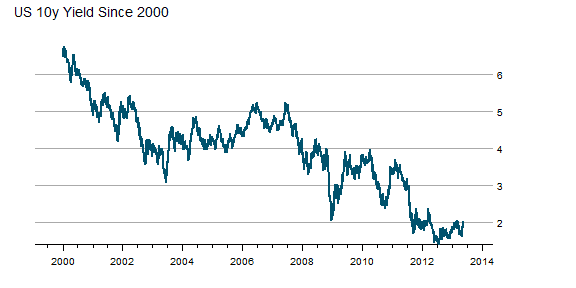Want to share your content on R-bloggers? click here if you have a blog, or here if you don't.

A quick glimpse at the US 10y Treasury Bond rate since 2000 seems benign with low volatility and a general downward trend.

```require(latticeExtra)
require(quantmod)

US10y <- getSymbols("^TNX", from = "2000-01-01", auto.assign = FALSE)[,
4]

asTheEconomist(xyplot(US10y, scales = list(y = list(rot = 1)),
main = "US 10y Yield Since 2000"))
```From TimelyPortfolio

However, if we plot the change in rate over 252 days or 1 year, we might wonder whether the rate is as benign as we initially surmised. Although this might seem like an acceptable intended consequence of the aggressive monetary easing by the US Fed, I fear that we will face the historically normal unintended consequences.

```p1 <- asTheEconomist(xyplot(US10y - lag(US10y, 252),
scales = list(y = list(rot = 0)), main = "US 10y Yield - Yearly Change"))
p1
```From TimelyPortfolio

And if we use some Vanguard US Bond Funds (vbmfx and vustx) as a proxy for the US bond market Approaching the Zero Bound - Bonds, we see that we are now in a market different than the last 4 years.

```require(directlabels)
require(reshape2)

getSymbols("VUSTX", from = "1990-01-01")

##  "VUSTX"

getSymbols("VBMFX", from = "1990-01-01")

##  "VBMFX"

bonds.tr <- merge(ROC(VUSTX[, 6], 250), ROC(VBMFX[,
6], 250))
colnames(bonds.tr) <- c("VanguardLongTsy", "VanguardTotBnd")
bond.funds.melt <- melt(as.data.frame(cbind(as.Date(index(bonds.tr)),
coredata(bonds.tr))), id.vars = 1)
colnames(bond.funds.melt) <- c("date", "fund", "totret250")
bond.funds.melt\$date <- as.Date(bond.funds.melt\$date)

p2 <- direct.label(xyplot(bonds.tr, screens = 1, ylim = c(-0.35,
0.35), scales = list(y = list(rot = 0)), col = theEconomist.theme()\$superpose.line\$col,
par.settings = theEconomist.theme(box = "transparent"),
lattice.options = theEconomist.opts(), xlab = NULL,
main = "Vanguard Bond Funds 250 Day Total Return"),
list("last.points", hjust = 1, cex = 1.2))
p2
```From TimelyPortfolio
```p3 <- asTheEconomist(horizonplot(totret250 ~ date |
fund, origin = 0, horizonscale = 0.05, data = bond.funds.melt,
strip = TRUE, strip.left = FALSE, par.strip.text = list(cex = 1.1),
layout = c(1, 2), main = "Vanguard Bond Funds 250 Day Total Return"))
p3
```From TimelyPortfolio
```# print(p2,position=c(0,0.4,1,1),more=TRUE)
# print(update(p3,main=NULL),position=c(0,0,1,0.5))
```

### Comparison to Japan

For some reference, let's look at a country getting quite a bit of attention lately in the press. We can add US yields to my favorite chart from Japan - JGB YieldsMore Lattice Charts. Most of the difference is in the short end of the curve where the US is still near the minimum since 2012 while Japan is near the max out to 7 years.

```url <- "http://www.mof.go.jp/english/jgbs/reference/interest_rate/"
filenames <- paste("jgbcme",c("","_2010","_2000-2009","_1990-1999","_1980-1989","_1974-1979"),".csv",sep="")

#load all data and combine into one jgb data.frame
for (i in 2:length(filenames)) {
}

#now clean up the jgb data.frame to make a jgb xts
jgb.xts <- as.xts(data.matrix(jgb[,2:NCOL(jgb)]),order.by=as.Date(jgb[,1]))
colnames(jgb.xts) <- paste0(gsub("X","JGB",colnames(jgb.xts)))

#get Yen from the Fed
#getSymbols("DEXJPUS",src="FRED")

xtsMelt <- function(data) {
require(reshape2)

#translate xts to time series to json with date and data
#for this behavior will be more generic than the original
#data will not be transformed, so template.rmd will be changed to reflect

#convert to data frame
data.df <- data.frame(cbind(format(index(data),"%Y-%m-%d"),coredata(data)))
colnames(data.df) = "date"
data.melt <- melt(data.df,id.vars=1,stringsAsFactors=FALSE)
colnames(data.melt) <- c("date","indexname","value")
#remove periods from indexnames to prevent javascript confusion
#these . usually come from spaces in the colnames when melted
data.melt[,"indexname"] <- apply(matrix(data.melt[,"indexname"]),2,gsub,pattern="[.]",replacement="")
return(data.melt)
#return(df2json(na.omit(data.melt)))

}

jgb.melt <- xtsMelt(jgb.xts["2012::",])
jgb.melt\$date <- as.Date(jgb.melt\$date)
jgb.melt\$value <- as.numeric(jgb.melt\$value)
jgb.melt\$indexname <- factor(
jgb.melt\$indexname,
levels = colnames(jgb.xts)
)
jgb.melt\$maturity <- as.numeric(
substr(
jgb.melt\$indexname,
4,
length( jgb.melt\$indexname ) - 4
)
)
jgb.melt\$country <- rep( "Japan", nrow( jgb.melt ))

#now get the US bonds from FRED
USbondssymbols <- paste0("DGS",c(1,2,3,5,7,10,20,30))

ust.xts <- xts()
for (i in 1:length( USbondssymbols ) ) {
ust.xts <- merge(
ust.xts,
getSymbols(
USbondssymbols[i],
auto.assign = FALSE,
src = "FRED"
)
)
}

ust.melt <- na.omit( xtsMelt( ust.xts["2012::",] ) )

ust.melt\$date <- as.Date(ust.melt\$date)
ust.melt\$value <- as.numeric(ust.melt\$value)
ust.melt\$indexname <- factor(
ust.melt\$indexname,
levels = colnames(ust.xts)
)
ust.melt\$maturity <- as.numeric(
substr(
ust.melt\$indexname,
4,
length( ust.melt\$indexname ) - 4
)
)
ust.melt\$country <- rep( "US", nrow( ust.melt ))

bonds.melt <- rbind( jgb.melt, ust.melt )

p4 <- xyplot(
value ~ date | maturity,
groups = country,
data = bonds.melt,
type = "l",
col = c(brewer.pal(9,"Blues"),brewer.pal(9,"Blues")),
layout = c( length( unique( bonds.melt\$maturity ) ), 1 ),
panel = function(x, y, subscripts, col, ...) {
panel.abline(
h = c(
sapply( by( y, bonds.melt[subscripts,"country"], summary ), min ),
sapply( by( y, bonds.melt[subscripts,"country"], summary ), max )
),
#if(!col){
col = col[1:length(unique(bonds.melt[subscripts,"country"]))]
#} else col = trellis.par.get()\$superpose.line\$col[1:length(unique(bonds.melt[subscripts,"country"]))]
)
panel.xyplot( x = x, y = y, subscripts = subscripts, col = col, ... )
panel.text(
x = x[ length(unique(x)) / 2],
y = mean(
c(
sapply( by( y, bonds.melt[subscripts,"country"], summary ), max ),
sapply( by( y, bonds.melt[subscripts,"country"], summary ), min )
)
),
labels = paste0(unique(bonds.melt\$maturity)[panel.number()],"y"),
cex = 0.8,
#font = 2,
col = "black",
)
},
scales = list(
x = list(
draw = FALSE
#tck = c(1,0),
#alternating = 1,
#at = min(bonds.melt\$date),
#labels = format(min(bonds.melt\$date),"%Y")
),
y = list( tck = c(1,0), lwd = c(0,1) )
),
strip = FALSE,
par.settings = list(axis.line = list(col = 0)),
xlab = NULL,
ylab = "Yield",
main = "JGB and US Yields by Maturity Since Jan 2012"
)
p4 <- p4 + layer(
panel.abline(
h = pretty(bonds.melt\$value,4),
lty = 3
)
)
p4
```From TimelyPortfolio

### Replicate

Gist source: https://gist.github.com/timelyportfolio/5665790